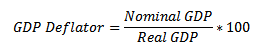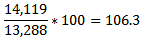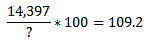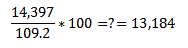Nominal vs. Real GDP or Prices, the use of the GDP deflator - FreeEconHelp.com, Learning Economics... Solved!

## 6/7/11

Nominal refers to a measurement of GDP given current prices.  Nominal prices are therefore the prices that we observe when we go shopping, or check out products on the internet.  Real GDP refers to measuring the GDP using past prices as a base.  Using real GDP or prices allows us to make comparisons across years without worrying about the effect of inflation.  For example, if we only look at nominal GDP numbers, we would see strong growth over time.  In order to find out how much GDP really grows, we need to use a tool called the GDP deflator.Great right?  But how do we figure this out?  We need to have nominal and real numbers
for GDP, and then we can figure out the deflator.  For example, imagine nominal GDP is 14,119 billion dollars, while real GDP is 13,288 billion dollars.  To get the deflator we need to divide the two:So the GDP deflator is 1.063.  This means that nominal GDP is about 6.3% higher than the true, or real GDP measure.

What if I give you nominal GDP and the deflator?  How will you get the real GDP measure?  Just use some simple algebra.  For example, say I gave you the nominal value of GDP as 14,397 billion dollars, with a GDP deflator of 109.2.  How would you solve this?  Set it up as follows:Multiply both sides by ?, and divide by 109.2 to get:So real GDP given these numbers is 13,184 billion dollars.

Remember:  Under what circumstances is the real GDP number equal to nominal GDP?  During the base year, or when the deflator is equal to zero!